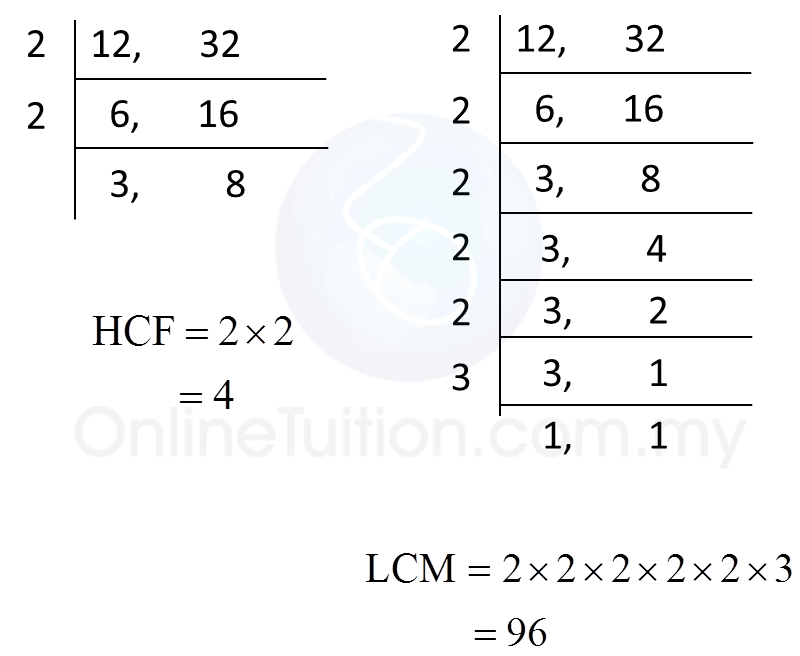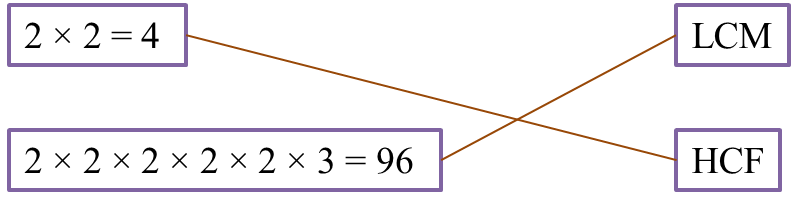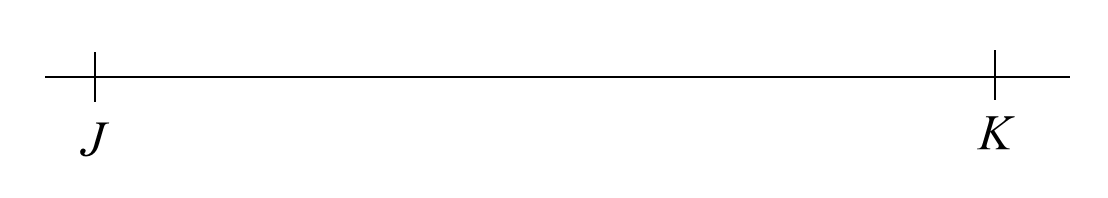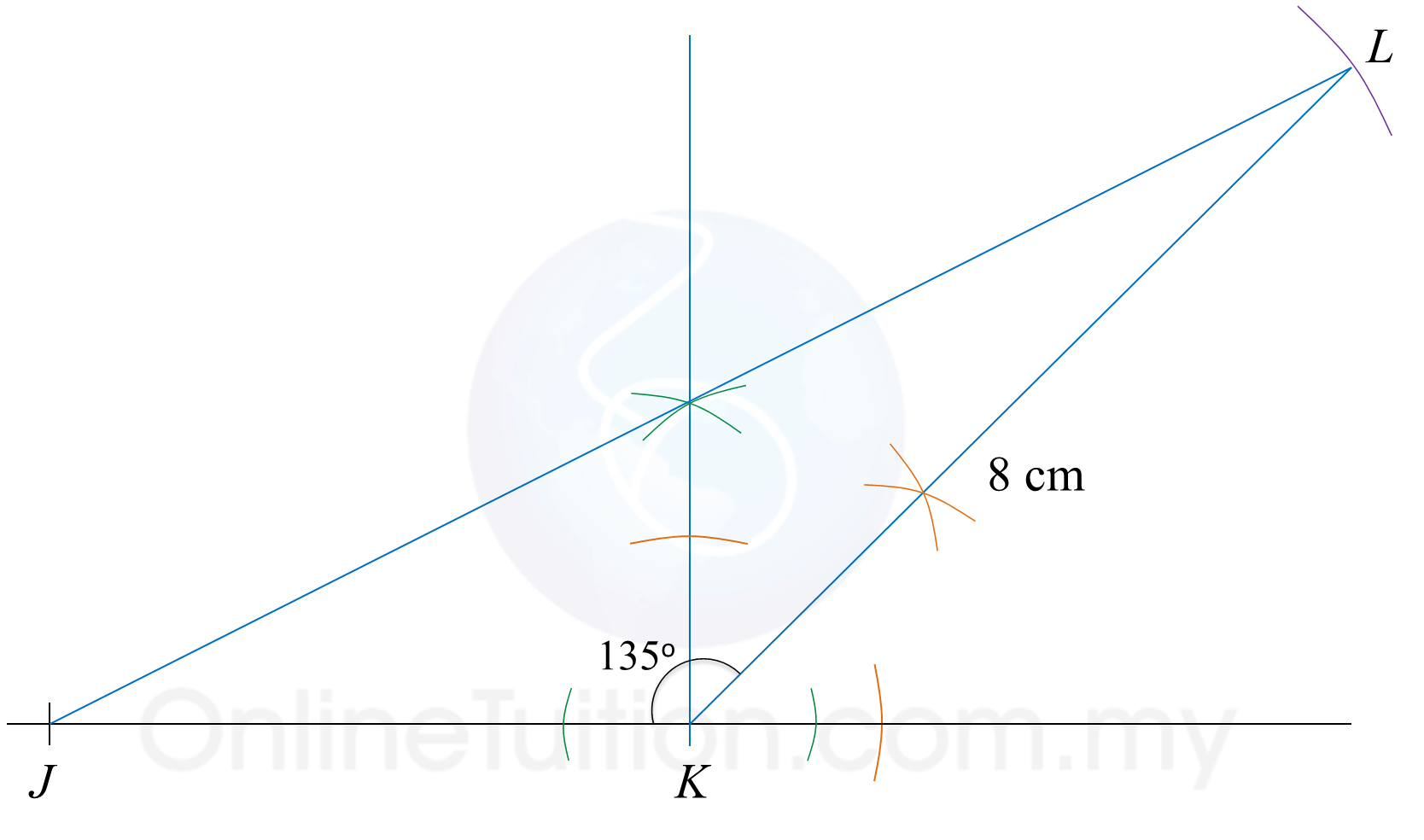# PT3 Mathematics 2017, Question 3

Question 3 (a):
(i) Diagram 3.1 shows one of the methods of finding the highest common factor (HCF) and the lowest common multiples (LCM) for 12 and 32.Match the correct answer.(ii) The difference between two prime numbers is 9.
Write the two prime numbers in the answer space.
(  ) – (  ) = 9

Solution:
(i)(ii)
( 11  ) – ( 2  ) = 9

Question 3 (b):
Diagram 3.2 in the answer space shows a straight line JK.
(i) Using only a ruler and a pair of compasses, construct triangle JKL with KL = 8 cm and ∠JKL = 135o. You may begin from the straight line JK provided in the answer space.(ii) Measure KJL by using a protractor.

Solution:
(i)(ii)
KJL = 26o

Question 3 (c):
List all the integer values of x which satisfy the following linear inequalities:
–1 ≤ 1 + 2x < 5

Solution:
–1 ≤ 1 + 2x < 5
–1 ≤ 1 + 2x   and   1 + 2x < 5
–2x ≤ 1 + 1   and   2x < 5 – 1
–2x ≤ 2    and   2x < 4
x ≥ –1   and   x < 2
x = –1, 0, 1, 2, … and   x = 1, 0, –1,  –2 …

Thus x = –1, 0, 1.